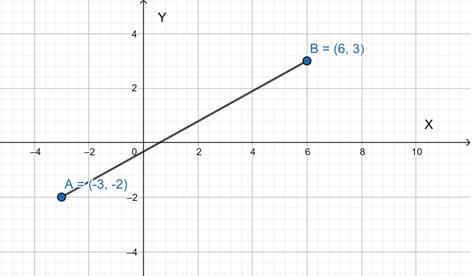# The function.### Precalculus: Mathematics for Calcu...

6th Edition
Stewart + 5 others
Publisher: Cengage Learning
ISBN: 9780840068071### Precalculus: Mathematics for Calcu...

6th Edition
Stewart + 5 others
Publisher: Cengage Learning
ISBN: 9780840068071

#### Solutions

Chapter 2.2, Problem 76E
To determine

## To find : The function.

Expert Solution

The function is y=5x913 .

### Explanation of Solution

Given information : The given coordinates are (3,2) and (6,3) .

Concept used : we must find out the slope of the equation. We can do this by the slope formula for the slope, m , is found by taking the change in y and a dividing it by the change of x .

Graph :Calculation :

m=y2y1x2x1=3+26+3=59

So,

yy1=m(xx1)y+2=59(x+3)y+2=5x9+159y=5x9+532y=5x9+563y=5x913

### Have a homework question?

Subscribe to bartleby learn! Ask subject matter experts 30 homework questions each month. Plus, you’ll have access to millions of step-by-step textbook answers!